# What Is the Binary Tree In Data Structure and How It Works?

Do you remember your school time, when we had started to learn to code? At that time we were store data in the array, which is common. Then we learned the hash table too.

But if you are doing graduation in computer science, and want to be an expert in the data structure, then you should learn about the binary tree. It’s a tree data structure in computer science. Similarly,

when you will learn about Binary trees and graphs, You will be able to solve a large amount of data. This post is to support you completely understand the binary tree in data structure and to remove confusion you may have about it.

Let’s begin.

## What Is the Binary Tree (BT)?

The binary tree structure is the same as a tree where a tree has leaves and each leaves connected through tree branches.

Similarly, the BT has nodes, and each node connected through edges to the next node; these nodes are also called a terminal node if they have no further any connected node.

The top position in the binary tree is called the parent/root. The node that connects through a root node is called a child node. And node is every data item present in a BT.

But what is important is that each binary tree root should have at most 2 nodes. However, any node who has no further any nodes we say them terminal node. Similarly, any node that joints through the same parent node can say sibling nodes.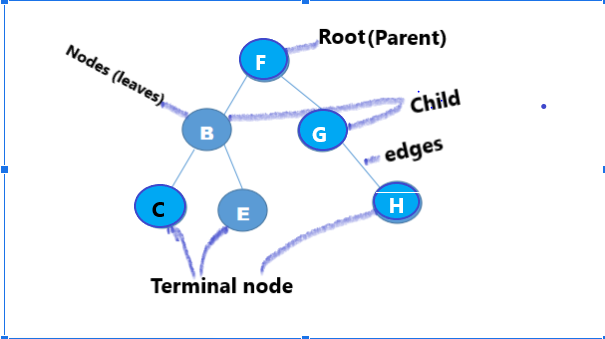Finally, the binary tree is a non-linear data structure. Before going into the depth of the topic, we should learn these important word meanings.

## Advantage of a binary tree

In any application, we need to store data and we need regular update and deletion or searching data. But when data become lengthy, it takes huge time for sorting. Then we need the binary tree method to reduce time.

1. Less time consuming rather Than stack and Ques.
2. It shows the hierarchies of data.
3. BT allow us easily insertion and deletion of data.
4. We can’t insert a duplicate value in a binary tree.

## Binary Tree terminology

1. Root: It is the first leaf in any binary tree.
2. Node: All the data or leaf in a BT is called a node.
3. Degree of a node: We can describe how many sub-tree of parent and child nodes are connected through a root.
We made a picture below so you can easily understand. where Root A has two degrees of the node.
• The first degree is number 1.
• Second Degree is number 2.Let’s see some other node

 Node Degree B 2 C 2 D 0
1. Non-terminal node: A node whose degree is not 0 and it should not be a root is called non-terminal node.1. Terminal node: A node has a 0 degree called terminal node.
2. Edges: It’s a line by which node connected by each other called edges.
Like: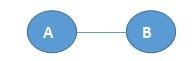1. Degree of tree: maximum degree of a node is a degree of tree. As above example node A degree is 3.
2. Depth of tree: It is the number of levels between root and terminal. There is a depth of a node tree is 2 in the image.1. Complete binary tree: complete binary tree should have all terminal nodes on the same level. As we shown above example.
2. Strictly binary tree: strictly binary tree’s every node should have either 0 or 2 node.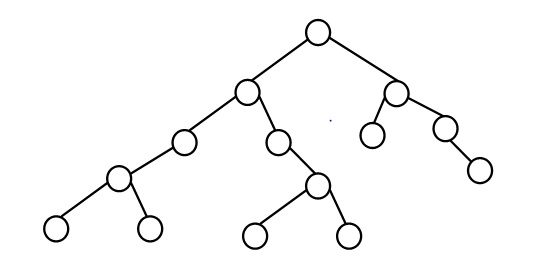## How to calculate the depth of any node?

There are two things to calculate in a binary tree, first is the depth of a BT (binary tree) and the second is the height of a BT.

1. Simply you have to count the longest path of BT’s edges for calculating the depth of a node.
Example 1.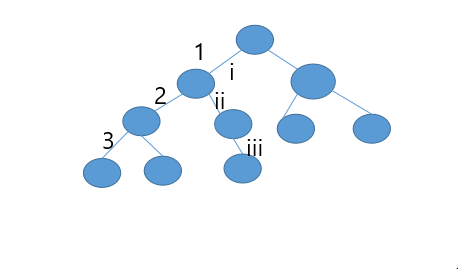2. In this example depth of a binary tree Is the total number of edges (3), thus the depth of BT= 3.
3. You can calculate the height of a BT=1+total number of edges.
Consider the above example we get.
Height of the binary tree=1+total number of edges (3) =1+3=4.
4. You can calculate the maximum number of nodes at every level in a binary tree by subtracting 1 from the number of levels in a binary tree.
Example 2. Calculate the maximum number of nodes in a BT.You can calculate by following this step.
Formula for calculate number of nodes at each level of BT = 2No. of level-1

 Apply a formula on the level 1. 2No. of level-1= 21-1=0 = 20 =1 node. Level 2. 2No. of level-1= 22-1 = 21 =2 node. Level 3. 2No. of level-1= 23-1=2 = 22 =4 node. Level 4. 2No. of level-1= 24-1=3 = 23 =8 node. Total number of nodes in a BT = T= 2d+1-1. where d is depth.Thus the total number of nodes in the above given BT is =23+1-1= 24-1. BT= 2*2*2*2-1=16-1=15 node.

You should also read binary search

## Binary tree traversal

A binary tree can use any of these operations on the data for searching, deleting, and insertion of any record.

1. Pre-order: First of all, the process visits the root/parent, then left child node and then right child node.
2. post-order: It first goes to the left child node, then right child node and finally parent node.
3. In-order. Firstly traversal visits the left child node, then the parent node and then the right child node.
S.No. Pre-Order Post-Order In-Order
The binary Tree image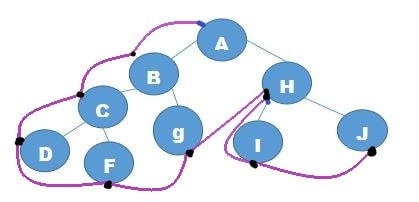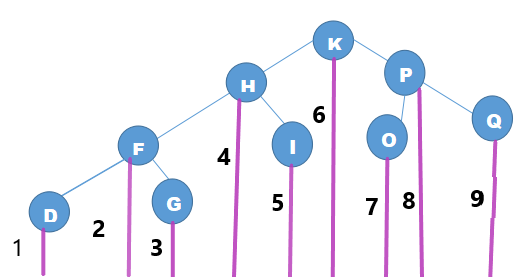1 In the above binary tree, you can see how the traversal process going on. It will show output as

K, H, F, D, G, I, P, O, Q.

 It will visit the first left-side node. And then right-side node after that visit their parent node.   Thus the output will be.  D, G, F, I, H, O, Q, P.
 Suppose all nodes are falling one by one. Then the output will show as  D, F, G, H, I, K, O, P, Q.

## How to search for data in the binary tree?

The binary tree is simply divided into 3 parts like Parent-node, left child node and right child node. Searching always starts from the root(parent node) node.

We compare that node with the root node whom we have to search. If it’s not that node that whom we want to discover then we move to the next node depending on the comparison.

If the result goes positive side, then we move to the “right-side” (right child-node) else we search in the left child node.

Then the binary tree will construct on the base of this algorithm.

Let’s see the BT search algorithm.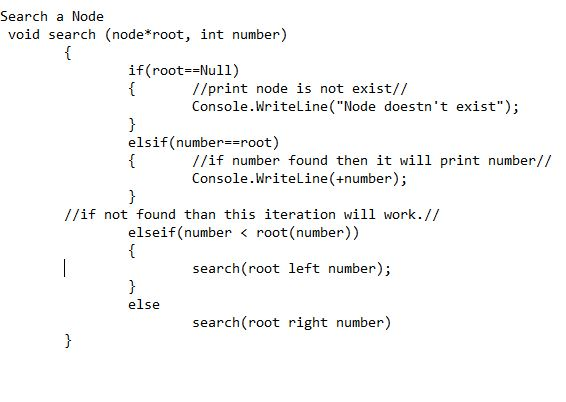Suppose we have to find 12 from given data, 25, 27, 28,26, 21, 22, 15, 14, 16.
And here root is 25 then.

First, it will compare to root if the root is equal to 12 then print 12.
If not equal to the root node, then it will check if 12 is less than the root node number then searching is done on the left side of the root.
And if 12 is greater than the root node than it will search on the right side of the root.

You can read java vs c# language.

## How to insert data in a binary tree?

We can insert data into a binary tree by traversing root to terminal node, you can take the above example, 25, 27, 28,26, 21, 22, 15, 14, 16. We have to add 29 in this data.

• Suppose root is 25 and check that 29 less than 25 or greater than 25.
Example of binary tree according to this data.• If greater than 25 then go to the right side and search that terminal node whose degree is 0.
• If found then add value. Let’s try to understand by an image.
 The complete binary tree will look like.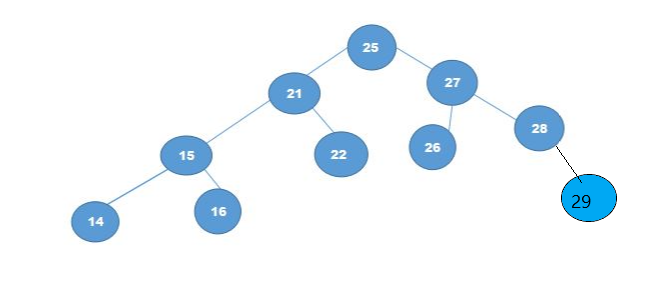Algorithm for insertion.

### How to delete a node in the binary tree?

First, we have to find a node which we have to delete as we did in insertion.

Like we have to delete 29 from above the BT.

1. Compare to the root, if 29 is greater than do right side searching.
2. The process continues until node founds.
3. If found then it might be 3 conditions,
• Node has no children: in this condition simply it deletes a node, as we showed in our example.
• While, node has one child: it deletes that node and replacing it to from child node
• Similarly, node has two children: If we delete 29 nodes, then we compare its child node that who is smaller, the small node will replace the large node.
And a bigger node will become child-node of the small node.
 Node has no children The Node has one child Node has two child-node.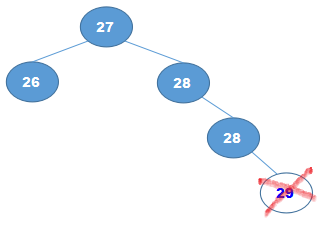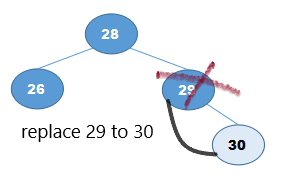“Node has two child nodes”, after the deletion process how it looks.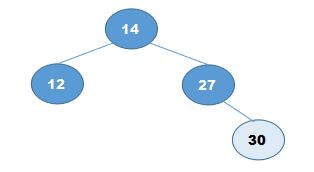## Summary

The binary tree is the most effective data searching technique, we can easily update our data structure. It’s based on the linear data structure.

It’s ideal for a large amount of data update.

We can use other data structures like arrays, a linked list, stack and queues but these all are used for the small amount of data.